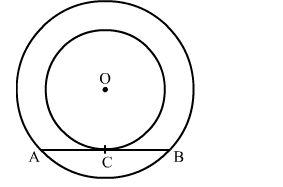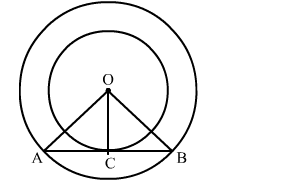# In the given figure, the chord AB of the larger of the two concentric circles, with centre O,Question:

In the given figure, the chord AB of the larger of the two concentric circles, with centre O, touches the smaller circle at C. Prove that AC = CB.Solution:

Construction:  Join OA, OC and OBWe know that the radius and tangent are perperpendular at their point of contact
∴ ∠OCA = ∠OCB = 90
Now, In △OCA and △OCB
∠OCA = ∠OCB = 90
OA = OB     (Radii of the larger circle)
OC = OC     (Common)
By RHS congruency
△OCA ≅ △OCB
∴ CA = CB